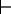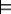#### 5.2.2.1 Bottom-Up Proof Procedure

The first proof procedure is a bottom-up proof procedure to derive logical consequences. It is called bottom-up as an analogy to building a house, where we build on top of the structure we already have. The bottom-up proof procedure builds on atoms that have already been established. It should be contrasted with a top-down approach, which starts from a query and tries to find definite clauses that support the query. Sometimes we say that a bottom-up procedure is forward chaining on the definite clauses, in the sense of going forward from what is known rather than going backward from the query.

The general idea is based on one rule of derivation, a generalized form of the rule of inference called modus ponens:

If "h←b1∧...∧bm" is a definite clause in the knowledge base, and each bi has been derived, then h can be derived.

This rule also covers the case when m=0; the bottom-up proof procedure can always immediately derive the atom at the head of a definite clause with no body.

1: Procedure DCDeductionBU(KB)
2:           Inputs
3:                     KB: a set of definite clauses
4:           Output
5:                     Set of all atoms that are logical consequences of KB
6:           Local
7:                     C is a set of atoms
8:           C←{}
9:           repeat
10:                     select "h←b1∧...∧bm" in KB where bi∈C for all i, and h ∉C
11:                     C←C∪{h}
12:           until no more definite clauses can be selected
13:           return C
Figure 5.3: Bottom-up proof procedure for computing consequences of KB

Figure 5.3 gives a procedure for computing the consequence set C of a set KB of definite clauses. Under this proof procedure, if g is an atom, KBg if g∈C at the end of the DCDeductionBU procedure. For a conjunction, KBg1∧...∧gk, if gi∈C for each i such that 0<i ≤ k.

Example 5.7: Suppose the system is given the knowledge base KB:
a←b∧c.
b←d∧e.
b←g∧e.
c←e.
d.
e.
f←a∧g.

One trace of the value assigned to C in the bottom-up procedure is

{}
{d}
{e,d}
{c,e,d}
{b,c,e,d}
{a,b,c,e,d}.

The algorithm terminates with C={a,b,c,e,d}. Thus, KBa, KBb, and so on.

The last rule in KB is never used. The bottom-up proof procedure cannot derive f or g. This is as it should be because a model of the knowledge base exists in which f and g are both false.

There are a number of properties that can be established for the proof procedure of Figure 5.3:

Soundness
Every atom in C is a logical consequence of KB. That is, if KBg then KBg. To show this, assume that an atom exists in C that is not a logical consequence of KB. If such an element exists, there must be a first element added to C that is not true in every model of KB. Suppose this is h, and suppose it is not true in model I of KB; h must be the first element generated that is false in I. Because h has been generated, there must be some definite clause in KB of the form h←b1∧...∧bm such that b1...bm are all in C (which includes the case where h is an atomic clause and so m=0). The bi are generated before h and so are all true in I. This clause's head is false in I, and its body is true in I; therefore, by the definition of truth of clauses, this clause is false in I. This is a contradiction to the fact that I is a model of KB. Thus, every element of C is a logical consequence of KB.
Complexity
The algorithm of Figure 5.3 halts, and the number of times the loop is repeated is bounded by the number of definite clauses in KB. This can be seen easily because each definite clause can only be used once. Thus, the time complexity of the preceding algorithm is linear in the size of the knowledge base if it can index the definite clauses so that the inner loop can be carried out in constant time.
Fixed Point
The final C generated in the algorithm of Figure 5.3 is called a fixed point because any further application of the rule of derivation does not change C. C is the minimum fixed point because there is no smaller fixed point.

Let I be the interpretation in which every atom in the minimum fixed point is true and every atom not in the minimum fixed point is false. To show that I must be a model of KB, suppose "h←b1∧...∧bm"∈KB is false in I. The only way this could occur is if b1,...,bm are in the fixed point, and h is not in the fixed point. By construction, the rule of derivation can be used to add h to the fixed point, a contradiction to it being the fixed point. Thus, there can be no definite clause in KB that is false in an interpretation defined by a fixed point. Thus, I is a model of KB.

Completeness
Suppose KBg. Then g is true in every model of KB, so it is true in the model I defined by the minimum fixed point, and so it is in C, and so KBg.

The model I defined above by the minimum fixed point is a minimum model in the sense that it has the fewest true propositions. Every other model must also assign the atoms in I to be true.

Non-deterministic Choice

In many AI programs, we want to separate the definition of a solution from how it is computed. Usually, the algorithms are non-deterministic, which means that there are choices in the program that are left unspecified. There are two sorts of non-determinism:

• Don't-care non-determinism is exemplified by the "select" in Figure 5.3. In this form of non-determinism, if one selection does not lead to a solution there is no point in trying any other alternatives. Don't-care non-determinism is used in resource allocation, where a number of requests occur for a limited number of resources, and a scheduling algorithm has to select who gets which resource at each time. The correctness is not affected by the selection, but efficiency and termination may be. When there is an infinite sequence of selections, a selection mechanism is fair if a request that is repeatedly available to be selected will eventually be selected. The problem of an element being repeatedly not selected is called starvation. We want to make sure that any selection is fair.
• Don't-know non-determinism is exemplified by the "choose" in Figure 5.4. Just because one choice did not lead to a solution does not mean that other choices will not work. Often we speak of an oracle that can specify, at each point, which choice will lead to a solution. Because our agent does not have such an oracle, it has to search through the space of alternate choices. Chapter 3 presents algorithms to search the space.

Don't-know non-determinism plays a large role in computational complexity theory. The class of P problems contains the problems solvable with time complexity polynomial in the size of the problem. The class of NP problems contains the problems that could be solved in polynomial time with an oracle that chooses the correct value at each time or, equivalently, if a solution is verifiable in polynomial time. It is widely conjectured that P≠NP, which would mean that no such oracle can exist. One great result of complexity theory is that the hardest problems in the NP class of problems are all equally complex; if one can be solved in polynomial time, they all can. These problems are NP-complete. A problem is NP-hard if it is at least as hard as an NP-complete problem.

In this book, we consistently use the term select for don't-care non-determinism and choose for don't-know non-determinism. In a non-deterministic procedure, we assume that an oracle makes an appropriate choice at each time. Thus, a choose statement will result in a choice that will led to success, or will fail if there are no such choices. A non-deterministic procedure may have multiple answers, where there are multiple choices that succeed, and will fail if there are no applicable choices. We can also explicitly fail a choice that should not succeed. The oracle is implemented by search.